Fraction Circles Template – Printable Fraction Circles – 11 Worksheets

Fraction Circles Template – Printable Fraction Circles

Halves, Thirds, Fourths, Fifths, Sixths, Sevenths, Eights, Ninths, Tenths, Elevenths, Twelfths

More fraction Circles Worksheets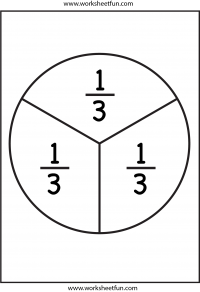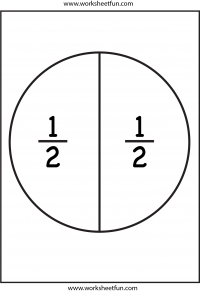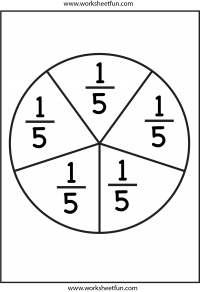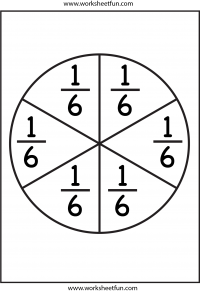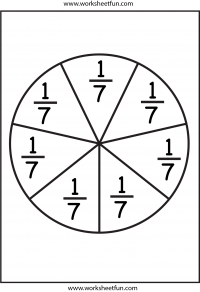More fraction Circles Worksheets

Fraction

Fraction Circles

Fraction Circles Template

Fraction Model

Fraction Subtraction

Fractions – Coloring

Fractions – Comparing

Fractions – Equivalent

Fractions – Halves